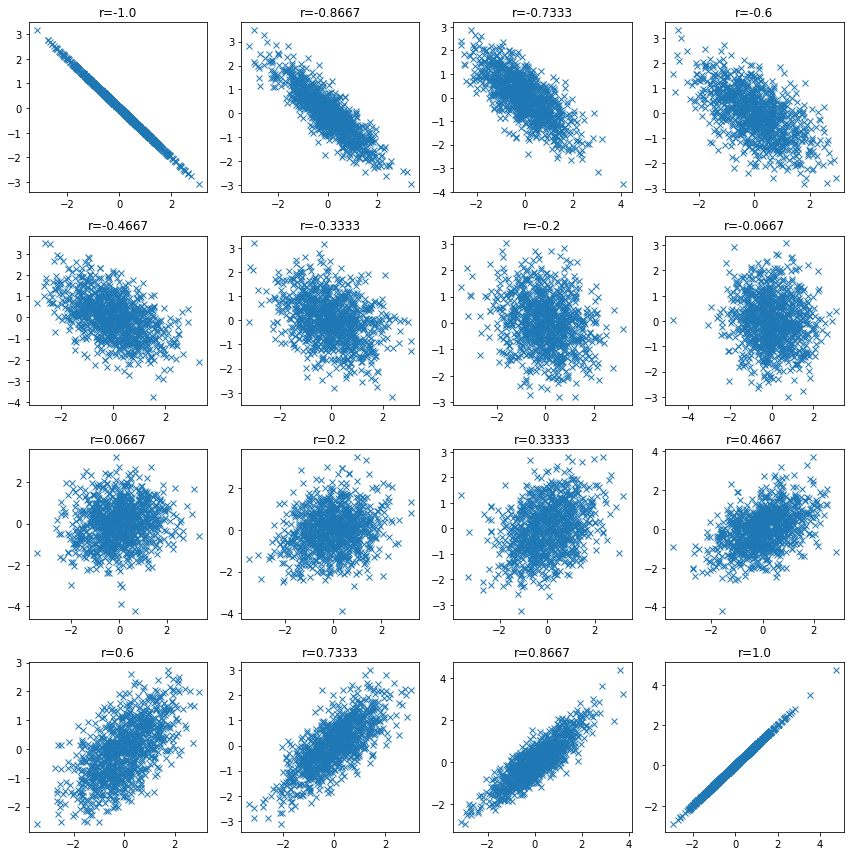# Correlation coefficient

Correlation coefficient measures the strength of a linear relationship between two data or random variables. It is an indicator that allows us to check whether there is a trend change of linear form between two variables, which can be expressed in the following equation.

$\frac{\Sigma_{i=1}^N (x_i - \bar{x})(y_i - \bar{y})}{\sqrt{\Sigma_{i=1}^N(x_i - \bar{x})^2 \Sigma_{i=1}^N(y_i - \bar{y})^2 }}$

It has the following properties

• 1 to less than 1
• If correlation coefficient is close to 1, $x$ increases → $y$ also increases
• The value of correlation coefficient does not change when $x, y$ are multiplied by a low number

## Calculate the correlation coefficient between two numerical columns

import numpy as np

np.random.seed(777)  # to fix random numbers

import matplotlib.pyplot as plt
import numpy as np

x = [xi + np.random.rand() for xi in np.linspace(0, 100, 40)]
y = [yi + np.random.rand() for yi in np.linspace(1, 50, 40)]

plt.figure(figsize=(5, 5))
plt.scatter(x, y)
plt.show()

coef = np.corrcoef(x, y)
print(coef)[[1.         0.99979848]
[0.99979848 1.        ]]


## Collectively compute the correlation coefficient between multiple variables

import seaborn as sns



sepal_lengthsepal_widthpetal_lengthpetal_widthspecies
05.13.51.40.2setosa
14.93.01.40.2setosa
24.73.21.30.2setosa
34.63.11.50.2setosa
45.03.61.40.2setosa

### Check the CORRELATION COEFFICIENCES between all variables

Using the iris dataset, let’s look at the correlation between variables.

df.corr().style.background_gradient(cmap="YlOrRd")

sepal_lengthsepal_widthpetal_lengthpetal_width
sepal_length1.000000-0.1175700.8717540.817941
sepal_width-0.1175701.000000-0.428440-0.366126
petal_length0.871754-0.4284401.0000000.962865
petal_width0.817941-0.3661260.9628651.000000

In the heatmap, it is hard to see where the correlation is highest. Check the bar chart to see which variables have the highest correlation with sepal_length.

df.corr()["sepal_length"].plot.bar(grid=True, ylabel="corr")## When correlation coefficient is low

Check the data distribution when the correlation coefficient is low and confirm that the correlation coefficient may be low even when there is a relationship between variables.

n_samples = 1000

plt.figure(figsize=(12, 12))
for i, ci in enumerate(np.linspace(-1, 1, 16)):
ci = np.round(ci, 4)

mean = np.array([0, 0])
cov = np.array([[1, ci], [ci, 1]])

v1, v2 = np.random.multivariate_normal(mean, cov, size=n_samples).T

plt.subplot(4, 4, i + 1)
plt.plot(v1, v2, "x")
plt.title(f"r={ci}")

plt.tight_layout()
plt.show()In some cases, there is a relationship between variables even if the correlation coefficient is low. We will try to create such an example, albeit a simple one.

import japanize_matplotlib
from sklearn import datasets

japanize_matplotlib.japanize()

n_samples = 1000
circle, _ = datasets.make_circles(n_samples=n_samples, factor=0.1, noise=0.05)
moon, _ = datasets.make_moons(n_samples=n_samples, noise=0.05)

corr_circle = np.round(np.corrcoef(circle[:, 0], circle[:, 1])[1, 0], 4)
plt.title(f"correlation coefficient={corr_circle}", fontsize=23)
plt.scatter(circle[:, 0], circle[:, 1])
plt.show()

corr_moon = np.round(np.corrcoef(moon[:, 0], moon[:, 1])[1, 0], 4)
plt.title(f"correlation coefficient={corr_moon}", fontsize=23)
plt.scatter(moon[:, 0], moon[:, 1])
plt.show()